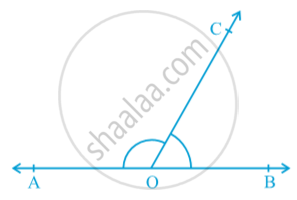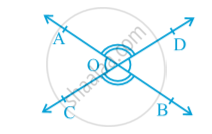# Pairs of Angles

#### text

Axiom :  If a ray stands on a line, then the sum of two adjacent angles so formed is 180°.The sum of two adjacent angles is 180°, then they are called a linear pair of angles.  It is given that ‘a ray stands on a line’. From this ‘given’, we have concluded that ‘the sum of two adjacent angles so formed is 180°’.
The ‘conclusion’ of Axiom as ‘given’ and the ‘given’ as the ‘conclusion’. So it becomes:
(A) If the sum of two adjacent angles is 180°, then a ray stands on a line (that is, the non-common arms form a line).
The Axiom  and statement (A) are in a sense the reverse of each others. We call each as converse of the other.

Axiom:   If the sum of two adjacent angles is 180°, then the non-common arms of the angles form a line. For obvious reasons, the two axioms above together is called the Linear Pair Axiom. Two lines intersect, the vertically opposite angles are equal.

#### theorem

Theorem:   If two lines intersect each other, then the vertically opposite angles are equal.
Proof : In the statement above, it is given that ‘two lines intersect each other’. So, let AB and CD be two lines intersecting at O as shown in Fig.They lead to two pairs of vertically opposite angles, namely,
(i) ∠ AOC and ∠ BOD (ii) ∠ AOD and ∠ BOC.
We need to prove that ∠ AOC = ∠ BOD and ∠ AOD = ∠ BOC.
Now, ray OA stands on line CD.
Therefore, ∠ AOC + ∠ AOD = 180° (Linear pair axiom) (1)
Can we write ∠ AOD + ∠ BOD = 180°        (2)
From (1) and (2), we can write
∠ AOC + ∠ AOD = ∠ AOD + ∠ BOD
This implies that ∠ AOC = ∠ BOD
Similarly, it can be proved that ∠AOD = ∠BOC.

If you would like to contribute notes or other learning material, please submit them using the button below.

### Shaalaa.com

Theorem: If two lines intersect each other, then the vertically opposite angles are equal. [00:18:41]
S
0%